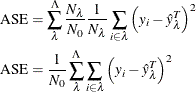Subtree Statistics

Subsections:

Statistics that are printed in the subtree tables are similar to the pruning statistics. There are two ways to calculate the subtree statistics: one is based on a scored data set (using the SCORE statement or the SAS DATA step score code that the CODE statement produces), and the other is based on the internal observation counts at each leaf of the tree. The two methods should provide identical results unless the target is missing.

Note: The per-observation and per-leaf methods of calculating the subtree statistics might not agree if the input data set contains observations that have a missing value for the target.

Decision Tree Per-Observation Methods

In scoring, whether you use the SCORE statement or you use the CODE statement with a SAS DATA step, each observation is assigned a posterior probability,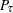, whereis a target level. These posterior probabilities are then used to calculate the subtree statistics of the final tree.

For a leaf, the posterior probability is the fraction of observations at that leaf that have the target level. That is, for leaf,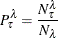When a record is scored, it is assigned to a leaf, and all posterior probabilities for that leaf are assigned along with it. Thus, for observation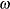assigned to leaf, the posterior probability is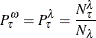The variable
continues to indicate the total number of observations in the input data set, andis the observation number (is used to prevent confusion with 0).

If a validation set is selected, the statistics are calculated separately for the validation set and for the training set. In addition, the per-observation validation posterior probabilities should be used. The validation posterior probabilities,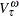, are the same as the posterior probabilities from the training set, but they are the fraction of the observations from the validation set that are in each target level,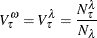where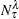and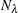are now observation counts from the validation set. For calculating the statistics on the validation set, the same equations can be used but substituting V for P where appropriate (for example,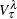for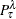).

Decision Tree Observationwise Entropy Statistic

The entropy at each observation is calculated from the posterior probabilities: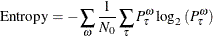Decision Tree Observationwise Gini Statistic

Like the entropy, the Gini statistic is also calculated from the posterior probabilities: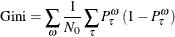Decision Tree Observationwise Misclassification Rate

The misclassification rate is the average number of incorrectly predicted observations in the input data set. Predictions are always based on the training set. Thus, each scored record’s predicted target level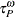is compared against the actual level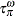: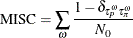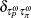is the Kronecker delta: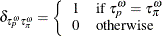Phrased slightly differently, the misclassification rate is the fraction of incorrectly predicted observations: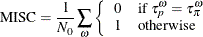Decision Tree Observationwise Sum of Squares Error

For the sum of squares error (SSE),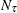predictions are made for every observation: that the correct posterior is 1 and that the incorrect posteriors is 0. Thus the SSE is as follows, whereis again the actual target level for observation: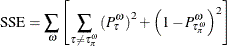Decision Tree Observationwise Average Square Error

The average square error (ASE) is simply the SSE divided by the number of predictions (there arepredictions per observation):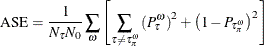Decision Tree Per-Leaf Methods

The subtree statistics that are calculated by PROC HPSPLIT are calculated per leaf. That is, instead of scanning through the entire data set, PROC HPSPLIT examines the proportions of observations at the leaves. Barring missing target values, which are not handled by the tree, the per-leaf and per-observation methods for calculating the subtree statistics are the same.

As with the per-observation method, observation counts N (,, and) can come from either the training set or the validation set. The growth subtree table always produces statistics from the training set. The pruning subtree table produces both sets of data if they are both present.

Unless otherwise marked, counts N can come from either set.

Decision Tree Leafwise Entropy Statistic

Because there areobservations on the leaf, entropy takes the following form: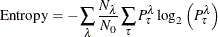Rephrased in terms of N, this becomes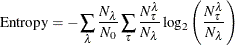Decision Tree Leafwise Gini Statistic

The Gini statistic is similar to entropy in its leafwise form: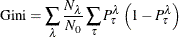Rephrased in terms of N, this becomes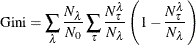Decision Tree Leafwise Misclassification Rate

Misclassification comes from the number of incorrectly predicted observations. Thus, it is necessary to count the proportion of observations at each leaf in each target level. Similar to the misprediction rate of an entire data set, the misprediction rate of a single leaf, is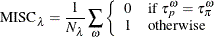where the summation is over the observations that arrive at a leaf.

All observations at a leaf are assigned the same prediction because they are all assigned the same leaf. Therefore, the summation reduces to simply the number of observations at leafthat have a target level other than the predicted target level for that leaf,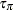. Thus,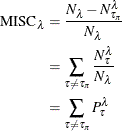whereisif the validation set is being examined.

Thus, for the entire data set, the misclassification rate is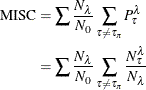where againisfor the validation set.

Decision Tree Leafwise Sum of Squares Error

The sum of squares error (SSE) is treated similarly to the misclassification rate. Each observation is assigned per-target posterior probabilitiesfrom the training data set. These are the predictions for the purpose of the SSE.

The observations at leafare then grouped by the observations’ target levels. Because each observation in the group has the same actual target level,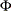, and because all observations on the same node are assigned the same posterior probabilities,, the per-observation SSE equation is identical: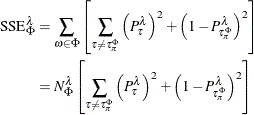Here, the posterior probabilitiesare from the training set, and the countsare from whichever data set is being examined.

Thus, the SSE equation for the leaf can be rephrased in terms of a further summation over the target levels: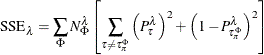So the SSE for the entire tree is then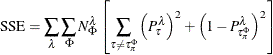Substituting the counts from the training set back in and using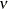to denote training set counts, this becomes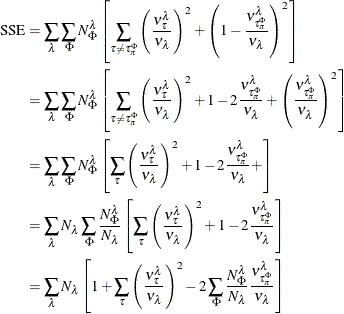In the rightmost inner summation,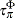is simply, the target level being summed over. This gives the final equivalent forms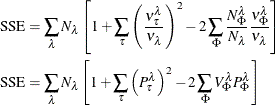whereand P are again counts and fraction, respectively, from the training set, and N and V are counts and fraction, respectively, from the validation set. (For example,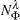is the number of observations on leafthat have target.)

If there is no validation set, the training set is used instead, and the equations simplify to the following (becauseis merely an index over target levels and can be renamed):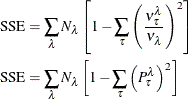Decision Tree Leafwise Average Square Error

Because the average square error (ASE) is simply the SSE divided by the number of predictions (there are, the number of target levels, predictions per observation), this becomes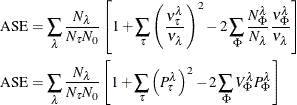Or, if only the training set is used: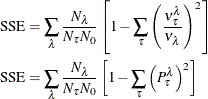Regression Tree Per-Observation Methods

Unlike a decision tree, regression trees model a continuous target. The predictions produced by a decision tree are based on the average of the partitioned space (the observations at the leaves).

As with decision trees, predictions come from the training set. The predicted value at leafis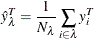where the summation is over the observations i within leaf, and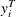is the value of the target variable at observation i within the training set.

Regression Tree Observationwise Sum of Squares Error

The observationwise sum of squares error (SSE) is the sum of squares of the difference between the observation’s value and the value that is predicted for that observation,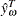, which is equal to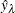whereis the leaf to which that observation has been assigned, as described in the equation in the preceding section. The SSE is then simply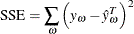Regression Tree Observationwise Average Square Error

The observationwise average square error (ASE) is simply the average of the observationwise square differences and is identical to the per-observation ASE: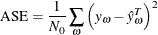Regression Tree Per-Leaf Methods

Regression Tree Leafwise Sum of Squares Error

The leafwise sum of squares error (SSE) is related to the variance within that leaf. The training set SSE is identical to the sum of the variances within the leaves: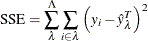Regression Tree Leafwise Average Square Error

The average square error (ASE) is simply the sum of weighted per-leaf average SSE’s. That is, the ASE is identical to the per-observation ASE: